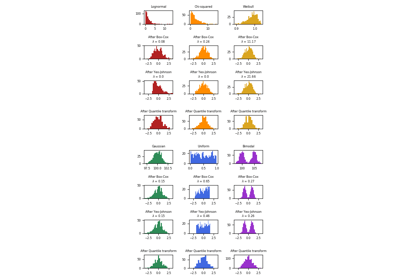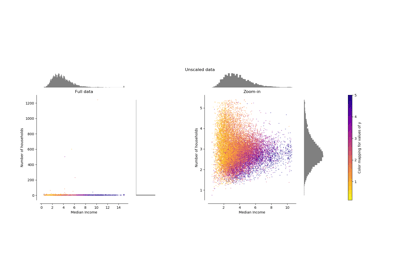/scikit-learn

# sklearn.preprocessing.PowerTransformer

`class sklearn.preprocessing.PowerTransformer(method=’yeo-johnson’, standardize=True, copy=True)` [source]

Apply a power transform featurewise to make data more Gaussian-like.

Power transforms are a family of parametric, monotonic transformations that are applied to make data more Gaussian-like. This is useful for modeling issues related to heteroscedasticity (non-constant variance), or other situations where normality is desired.

Currently, PowerTransformer supports the Box-Cox transform and the Yeo-Johson transform. The optimal parameter for stabilizing variance and minimizing skewness is estimated through maximum likelihood.

Box-Cox requires input data to be strictly positive, while Yeo-Johnson supports both positive or negative data.

By default, zero-mean, unit-variance normalization is applied to the transformed data.

Read more in the User Guide.

Parameters: `method : str, (default=’yeo-johnson’)` The power transform method. Available methods are: ‘yeo-johnson’ , works with positive and negative values ‘box-cox’ , only works with strictly positive values `standardize : boolean, default=True` Set to True to apply zero-mean, unit-variance normalization to the transformed output. `copy : boolean, optional, default=True` Set to False to perform inplace computation during transformation. `lambdas_ : array of float, shape (n_features,)` The parameters of the power transformation for the selected features.

`power_transform`
Equivalent function without the estimator API.
`QuantileTransformer`
Maps data to a standard normal distribution with the parameter `output_distribution=’normal’`.

#### Notes

NaNs are treated as missing values: disregarded in fit, and maintained in transform.

For a comparison of the different scalers, transformers, and normalizers, see examples/preprocessing/plot_all_scaling.py.

#### References

  (1, 2) I.K. Yeo and R.A. Johnson, “A new family of power transformations to improve normality or symmetry.” Biometrika, 87(4), pp.954-959, (2000).
  (1, 2) G.E.P. Box and D.R. Cox, “An Analysis of Transformations”, Journal of the Royal Statistical Society B, 26, 211-252 (1964).

#### Examples

```>>> import numpy as np
>>> from sklearn.preprocessing import PowerTransformer
>>> pt = PowerTransformer()
>>> data = [[1, 2], [3, 2], [4, 5]]
>>> print(pt.fit(data))
PowerTransformer(copy=True, method='yeo-johnson', standardize=True)
>>> print(pt.lambdas_)
[1.38668178e+00 5.93926346e-09]
>>> print(pt.transform(data))
[[-1.31616039 -0.70710678]
[ 0.20998268 -0.70710678]
[ 1.1061777   1.41421356]]
```

#### Methods

 `fit`(X[, y]) Estimate the optimal parameter lambda for each feature. `fit_transform`(X[, y]) `get_params`([deep]) Get parameters for this estimator. `inverse_transform`(X) Apply the inverse power transformation using the fitted lambdas. `set_params`(**params) Set the parameters of this estimator. `transform`(X) Apply the power transform to each feature using the fitted lambdas.
`__init__(method=’yeo-johnson’, standardize=True, copy=True)` [source]
`fit(X, y=None)` [source]

Estimate the optimal parameter lambda for each feature.

The optimal lambda parameter for minimizing skewness is estimated on each feature independently using maximum likelihood.

Parameters: `X : array-like, shape (n_samples, n_features)` The data used to estimate the optimal transformation parameters. `y : Ignored` `self : object`
`get_params(deep=True)` [source]

Get parameters for this estimator.

Parameters: `deep : boolean, optional` If True, will return the parameters for this estimator and contained subobjects that are estimators. `params : mapping of string to any` Parameter names mapped to their values.
`inverse_transform(X)` [source]

Apply the inverse power transformation using the fitted lambdas.

The inverse of the Box-Cox transformation is given by:

```if lambda == 0:
X = exp(X_trans)
else:
X = (X_trans * lambda + 1) ** (1 / lambda)
```

The inverse of the Yeo-Johnson transformation is given by:

```if X >= 0 and lambda == 0:
X = exp(X_trans) - 1
elif X >= 0 and lambda != 0:
X = (X_trans * lambda + 1) ** (1 / lambda) - 1
elif X < 0 and lambda != 2:
X = 1 - (-(2 - lambda) * X_trans + 1) ** (1 / (2 - lambda))
elif X < 0 and lambda == 2:
X = 1 - exp(-X_trans)
```
Parameters: `X : array-like, shape (n_samples, n_features)` The transformed data. `X : array-like, shape (n_samples, n_features)` The original data
`set_params(**params)` [source]

Set the parameters of this estimator.

The method works on simple estimators as well as on nested objects (such as pipelines). The latter have parameters of the form `<component>__<parameter>` so that it’s possible to update each component of a nested object.

Returns: self
`transform(X)` [source]

Apply the power transform to each feature using the fitted lambdas.

Parameters: `X : array-like, shape (n_samples, n_features)` The data to be transformed using a power transformation. `X_trans : array-like, shape (n_samples, n_features)` The transformed data.

## Examples using `sklearn.preprocessing.PowerTransformer`Map data to a normal distributionCompare the effect of different scalers on data with outliers